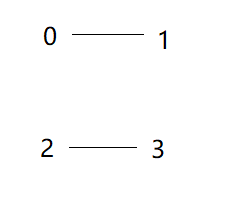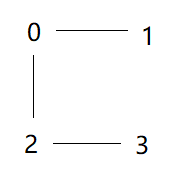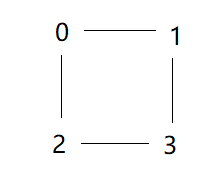# Leetcode之并查集专题-765. 情侣牵手（Couples Holding Hands）

Leetcode之并查集专题-765. 情侣牵手（Couples Holding Hands）

N 对情侣坐在连续排列的 2N 个座位上，想要牵到对方的手。 计算最少交换座位的次数，以便每对情侣可以并肩坐在一起。 次交换可选择任意两人，让他们站起来交换座位。

输入: row = [0, 2, 1, 3]



输入: row = [3, 2, 0, 1]



1. len(row) 是偶数且数值在 [4, 60]范围内。
2. 可以保证row 是序列 0...len(row)-1 的一个全排列。第一步里，f=0,f=0,f=2,f=2;class Solution {
int[] f;
public int minSwapsCouples(int[] row) {
int len = row.length;
int res = 0;
f = new int[len];
for (int i = 0; i < row.length-1; i+=2) {
f[i] = i;
f[i+1] = i;
}
for (int i = 0; i < row.length-1; i+=2) {
int first = row[i];
int second = row[i+1];
int father1 = getFather(first);
int father2 = getFather(second);
if(father1!=father2){
f[father2] = father1;
}else{
res++;
}
}
return (len/2) - res;
}

private int getFather(int i) {
if(f[i]==i){
return i;
}else{
return getFather(f[i]);
}
}
}

posted @ 2019-09-12 00:23  秦羽纶  阅读(585)  评论(0编辑  收藏